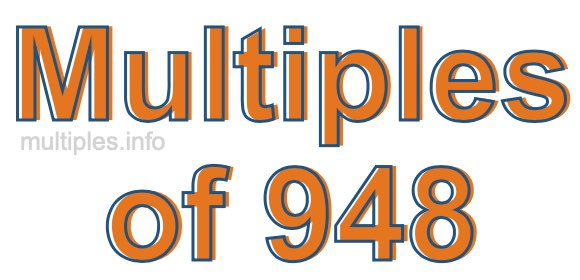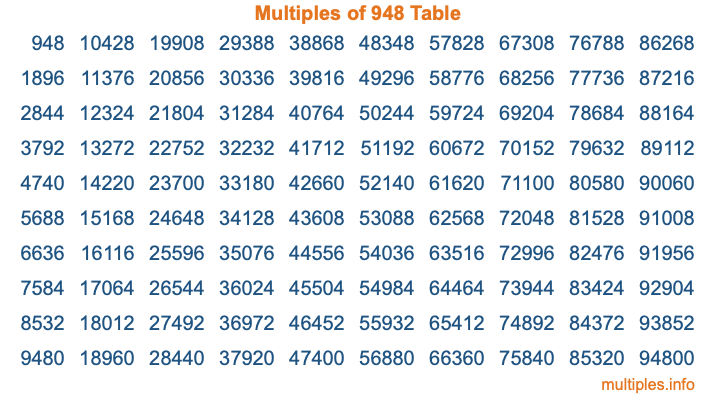Multiples of 948Welcome to the Multiples of 948 page. Here we will first teach you everything you will ever need to know about the multiples of 948, and then give you a study guide summary of everything we taught you to make sure you remember it all. Use this page to look up facts and learn information about the multiples of 948. This page will make you a multiples of nine hundred forty-eight expert!

Definition of Multiples of 948
Multiples of 948 are all the numbers that when divided by 948 equal an integer. Each of the multiples of 948 are called a multiple. A multiple of 948 is created by multiplying 948 by an integer.

Therefore, to create a list of multiples of 948, you start with 1 multiplied by 948, then 2 multiplied by 948, then 3 multiplied by 948, and so on for as long as you want. Thus, the list of the first five multiples of 948 is 948, 1896, 2844, 3792, and 4740. To see a larger list of multiples of 948, see the printable image of Multiples of 948 further down on this page. We also have a category where you can choose any nth multiple of 948.

Multiples of 948 Checker
The Multiples of 948 Checker below checks to see if any number of your choice is a multiple of 948. In other words, it checks to see if there is any number (integer) that when multiplied by 948 will equal your number. To do that, we divide your number by 948. If the the quotient is an integer, then your number is a multiple of 948.

Is  a multiple of 948?

Least Common Multiple of 948 and ...
A Least Common Multiple (LCM) is the lowest multiple that two or more numbers have in common. This is also called the smallest common multiple or lowest common multiple and is useful to know when you are adding our subtracting fractions. Enter one or more numbers below (948 is already entered) to find the LCM.

Check out our LCM Calculator if you need more details about the Least Common Multiple or if you need the LCM for different numbers for adding and subtraction fractions.

nth Multiple of 948
As we stated above, 948 is the first multiple of 948, 1896 is the second multiple of 948, 2844 is the third multiple of 948, and so on. Enter a number below to find the nth multiple of 948.

th multiple of 948

Multiples of 948 vs Factors of 948
948 is a multiple of 948 and a factor of 948, but that is where the similarities end. All postive multiples of 948 are 948 or greater than 948. All positive factors of 948 are 948 or less than 948.

Below is the beginning list of multiples of 948 and the factors of 948 so you can compare:

Multiples of 948: 948, 1896, 2844, 3792, 4740, etc.

Factors of 948: 1, 2, 3, 4, 6, 12, 79, 158, 237, 316, 474, 948

As you can see, the multiples of 948 are all the numbers that you can divide by 948 to get a whole number. The factors of 948, on the other hand, are all the whole numbers that you can multiply by another whole number to get 948.

It's also interesting to note that if a number (x) is a factor of 948, then 948 will also be a multiple of that number (x).

Multiples of 948 vs Divisors of 948
The divisors of 948 are all the integers that 948 can be divided by evenly. Below is a list of the divisors of 948.

Divisors of 948: 1, 2, 3, 4, 6, 12, 79, 158, 237, 316, 474, 948

The interesting thing to note here is that if you take any multiple of 948 and divide it by a divisor of 948, you will see that the quotient is an integer.

Multiples of 948 Table
Below is an image of the first 100 multiples of 948 in a table. The table is in chronological order, column by column. The first column has the first ten multiples of 948, the second column has the next ten multiples of 948, and so on.The Multiples of 948 Table is also referred to as the 948 Times Table or Times Table of 948. You are welcome to print out our table for your studies.

Negative Multiples of 948
Although not often discussed or needed in math, it is worth mentioning that you can make a list of negative multiples of 948 by multiplying 948 by -1, then by -2, then by -3, and so on, to get the following list of negative multiples of 948:

-948, -1896, -2844, -3792, -4740, etc.

Multiples of 948 Summary
Below is a summary of important Multiples of 948 facts that we have discussed on this page. To retain the knowledge on this page, we recommend that you read through the summary and explain to yourself or a study partner why they hold true.

There are an infinite number of multiples of 948.

A multiple of 948 divided by 948 will equal a whole number.

948 divided by a factor of 948 equals a divisor of 948.

The nth multiple of 948 is n times 948.

The largest factor of 948 is equal to the first positive multiple of 948.

948 is a multiple of every factor of 948.

948 is a multiple of 948.

A multiple of 948 divided by a divisor of 948 equals an integer.

948 divided by a divisor of 948 equals a factor of 948.

Any integer times 948 will equal a multiple of 948.

Multiples of a Number
Here you can get the multiples of another number, all with the same attention to detail as we did for multiples of 948 on this page.

Multiples of
Multiples of 949
Did you find our page about multiples of nine hundred forty-eight educational? Do you want more knowledge? Check out the multiples of the next number on our list!